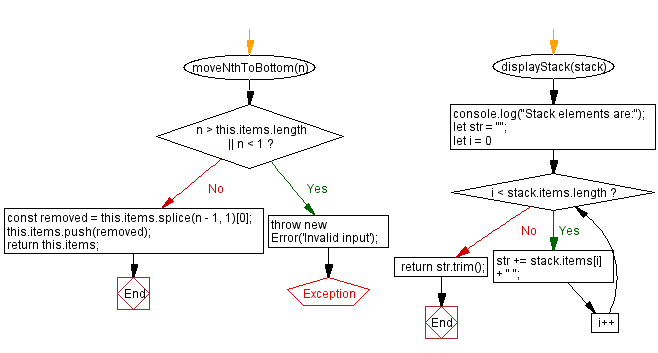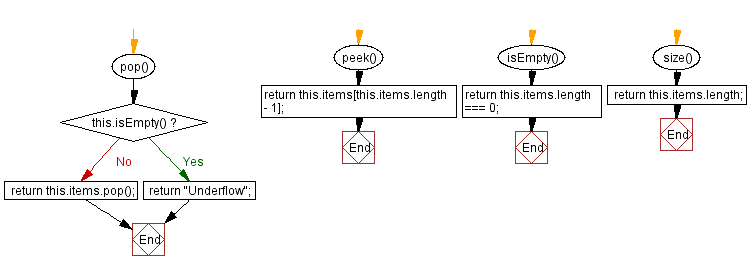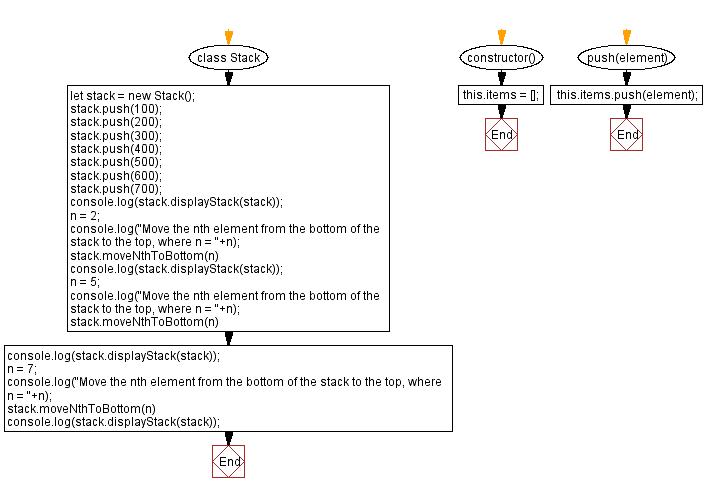# JavaScript Exercises: Move the nth element from the top of the stack to the top

## JavaScript Stack: Exercise-18 with Solution

Write a JavaScript program to implement a stack and move the nth element from the top of the stack to the top.

Sample Solution:

JavaScript Code:

``````class Stack {
constructor() {
this.items = [];
}

push(element) {
this.items.push(element);
}
pop() {
if (this.isEmpty()) {
return "Underflow";
}
return this.items.pop();
}
peek() {
return this.items[this.items.length - 1];
}
isEmpty() {
return this.items.length === 0;
}
size() {
return this.items.length;
}
moveNthToTop(n) {
if (this.items.length == 0) {
return this.items;
}
if (n > this.items.length) {
return this.items;
}
let tempStack = [];
for (let i = 0; i < n - 1; i++) {
tempStack.push(this.items.pop());
}
let nthElement = this.items.pop();
while (tempStack.length > 0) {
this.items.push(tempStack.pop());
}
tempStack.push(nthElement);
while (this.items.length > 0) {
tempStack.push(this.items.pop());
}
while (tempStack.length > 0) {
this.items.push(tempStack.pop());
}
return this.items;
}
displayStack(stack) {
console.log("Stack elements are:");
let str = "";
for (let i = 0; i < stack.items.length; i++)
str += stack.items[i] + " ";
return str.trim();
}
}

let stack = new Stack();
stack.push(100);
stack.push(200);
stack.push(300);
stack.push(400);
stack.push(500);
stack.push(600);
stack.push(700);
console.log(stack.displayStack(stack));
n = 2;
console.log("Move the nth element from the top of the stack to the top, where n = "+n);
stack.moveNthToTop(n)
console.log(stack.displayStack(stack));
n = 5;
console.log("Move the nth element from the top of the stack to the top, where n = "+n);
stack.moveNthToTop(n)
console.log(stack.displayStack(stack));
n = 7;
console.log("Move the nth element from the top of the stack to the top, where n = "+n);
stack.moveNthToTop(n)
console.log(stack.displayStack(stack));
```
```

Sample Output:

```Stack elements are:
100 200 300 400 500 600 700
Move the nth element from the top of the stack to the top, where n = 2
Stack elements are:
100 200 300 400 500 700 600
Move the nth element from the top of the stack to the top, where n = 5
Stack elements are:
100 200 400 500 700 600 300
Move the nth element from the top of the stack to the top, where n = 7
Stack elements are:
200 400 500 700 600 300 100
```

Flowchart:Live Demo:

See the Pen javascript-stack-exercise-18 by w3resource (@w3resource) on CodePen.

Improve this sample solution and post your code through Disqus

Stack Previous: Nth element from the bottom of the stack.
Stack Exercises Next: Move the nth element from the bottom of the stack to the top.

What is the difficulty level of this exercise?

Test your Programming skills with w3resource's quiz.

﻿